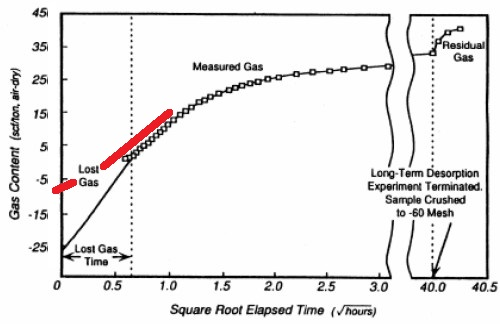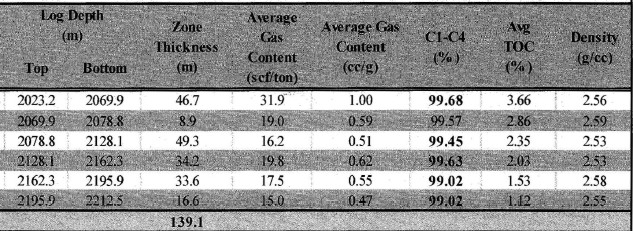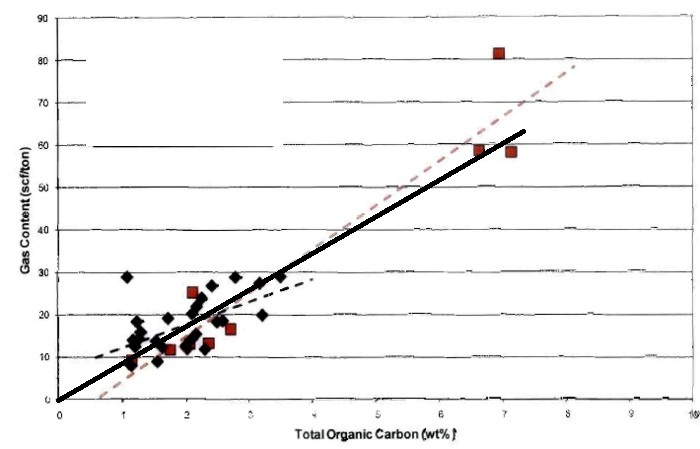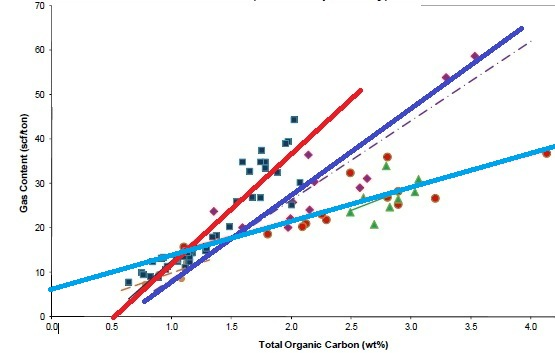Shale gas BASICS
Shale is a fine-grained, clastic sedimentary rock composed of mud that is a mix of clay minerals and tiny fragments (silt-sized particles) of other minerals, especially quartz, dolomite, and calcite. The ratio of clay to other minerals varies. Shale is characterized by breaks along thin laminations, parallel to the bedding. Mudstones are similar in composition but do not usually show layering within the zone.

The distinguishing characteristic of gas shales is that they have adsorbed gas, just like coal beds. They also have free gas in porosity, unlike coal, which has virtually no macro-porosity. The adsorbed gas is proportional to the organic content of the shale. Free gas is proportional to the effective porosity and gas saturation in the pores.Sorption isothermsSorption isotherms indicate the maximum volume of methane that a gas shale can store under equilibrium conditions at a given pressure and temperature. The direct method of determining sorption isotherms involves drilling and cutting core that is immediately placed in canisters, followed by measurements of the gas volume (Gc) evolved from the shale over time.

<== Sorption isotherms for a gas shale, as measured
(Note that the lost gas estimate is absurdly optimistic
and doesn't follow the measured trend toward zero time)

When the sample no longer evolves gas, it is crushed and the residual gas is measured. A detailed description of the lab measurement of adsorbed gas is provided in the CBM Chapter.

Gas content (Gc) results are usually given as scf/ton or cc/gram, as shown in the example lab report below. Multiply Gc in cc/gram by 32.18 to get Gc in scf/ton.Example of Gas Content as measured in the lab in a shale gas interval.GAS CONTENT  Versus TOC
TOC derived from log analysis models are widely used as a guide to the quality of gas shales. Using correlations of lab measured TOC and gas content (Gc). We can use log analysis derived TOC values to predict Gc, which can then be summed over the interval and converted to adsorbed gas in place. Sample correlations are shown below.Crossplots of TOC versus Gc for Tight Gas / Shale Gas examples. Note the large variation in Gc versus TOC for different rocks, and that the correlations are not always very strong. These data sets are from core samples; cuttings give much worse correlations. The fact that some best fit lines do not pass through the origin suggests systematic errors in measurement or recovery and preservation techniques, and erroneous lost gas estimates.

Gas content from correlation of core analysis data:
1: Gc = KG11 * TOC%

Where:
Gc = gas content (scf/ton)
TOC% = total organic carbon (weight percent)
KG11 = gas parameter, varies between 5 and 15SHALE  Gas In Place - adsorbed
Gas in place calculations in gas shales are done in two parts: adsorbed gas and free gas.

Adsorbed gas in place is calculated from the actual gas content found in the lab or from a correlation between TOC and gas content (generated from lab measured data). Examples of both data sources are shown below..

Gas in place is derived from:
2: GIPadsorb = KG6 * Gc * DENS * THICK * AREA

Where:
GIPadsorb = gas in place (Bcf)
Gc = sorbed gas from lab measured isotherm (scf/ton)
DENS = layer density from log or lab measurement (g/cc)
THICK = layer thickness (feet)
AREA = spacing unit area (acres)
KG6 = 1.3597*10^-6

If AREA = 640 acres, then GIP = Bcf/Section (= Bcf/sq.mile)
Multiply meters by 3.281 to obtain thickness in feet.
Multiply Gc in cc/gram by 32.18 to get Gc in scf/ton.COMMENTS
Typical shale densities are in the range of 2.20 to 2.60 g/cc.

Recoverable gas can be estimated by using the sorption curve at abandonment pressure (Ga) and replacing Gc in Equation 1 with (Gc - Ga).

"ground truth".SHALE  Gas In Place - FREE GAS
Free gas in place is calculated from the usual volumetric equations using the porosity and water saturations developed by the kerogen corrected log analysis model:
22: Bg =  (Ps * (Tf + KT2)) / (Pf * (Ts + KT2)) * ZF

23: GIPfree = KV4 * (1 - Qnc) * PHIe * (1 - Sw) * THICK *  AREA / Bg
24: GIPtotal = GIPadsorb + GIPfree

Where:
AREA = reservoir area (acres)
Bg = gas formation volume factor (fractional)
GIPfree = original free gas in place (Bcf)
GIPtotal = total gas in place (Bcf)
PHIe = effective porosity (fractional)
Sw = water saturation in un-invaded zone (fractional)
THICK = layer thickness (feet)
Pf = formation pressure (psi)

Ps = surface pressure (psi)
Tf = formation temperature ('F)
Ts = surface temperature ('F)
ZF = gas compressibility factor (fractional)
KT2 = 460'F
KV4 = 0.000 043 560
Qnc = fraction of gas that is non-combustible (CO2, N2,etc)

If AREA = 640 acres, then GIP = Bcf/Section (= Bcf/sq.mile)
Multiply meters by 3.281 to obtain thickness in feet.

Page Views ---- Since 01 Jan 2018
Copyright 2023 by Accessible Petrophysics Ltd.
CPH Logo, "CPH", "CPH Gold Member", "CPH Platinum Member", "Crain's Rules", "Meta/Log", "Computer-Ready-Math", "Petro/Fusion Scripts" are Trademarks of the Author